How Cheenta works to ensure student success?
Explore the Back-Story

# Test of Mathematics Solution Subjective 127 -Graphing relations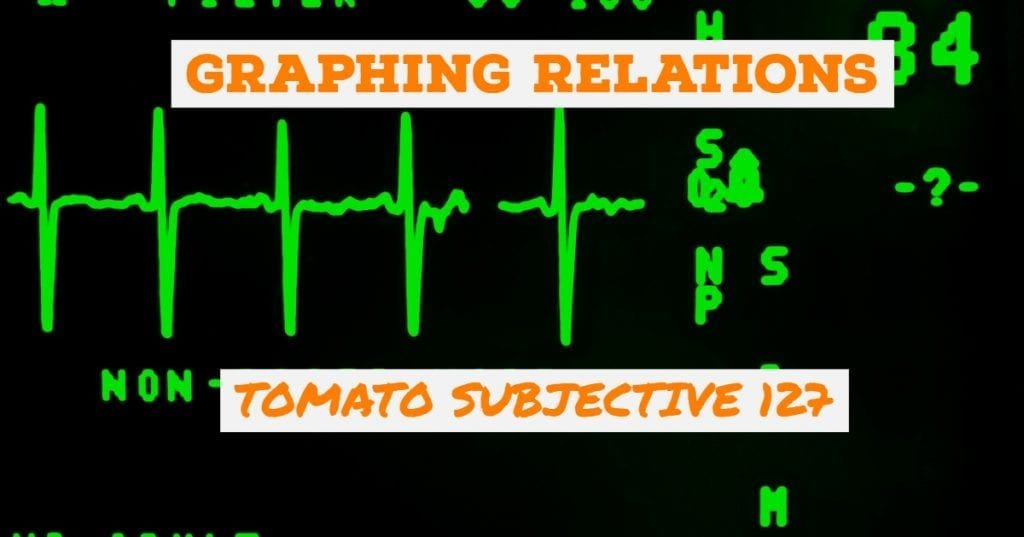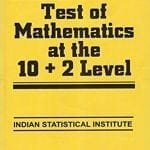This is a Test of Mathematics Solution Subjective 127 (from ISI Entrance). The book, Test of Mathematics at 10+2 Level is Published by East West Press. This problem book is indispensable for the preparation of I.S.I. B.Stat and B.Math Entrance.

## Problem

Find all (x, y) such that sin x + sin y = sin (x+y) and |x| + |y| = 1

## Discussion

|x| + |y| =1 is easier to plot. We have to treat the cases separately.

• First quadrant: x +y = 1
• Second quadrant: -x + y = 1 (since |x| = -x when x is negative)
• Fourth Quadrant: x - y =1Now we work on sin x + sin y = sin (x + y).

This implies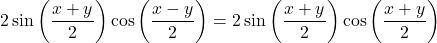. Hence we have two possibilities:

•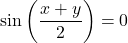OR
•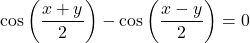or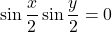The above situations can happen when whenor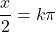or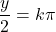, where k is any integer.

Thus we need to plot the class of lines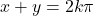,and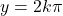, and consider the intersection points of these lines with the graph of |x| + |y| = 1.Clearly only for k=0, such intersection points can be found.

Hence required points are (0,1), (0,-1), (1,0), (-1,0), (1/2, -1/2), (-1/2, 1/2).

## Chatuspathi

• What is this topic: Graphing
• What are some of the associated concept: Trigonometric Identities
• Where can learn these topics: I.S.I. & C.M.I. Entrance Course of Cheenta, discusses these topics in the ‘Calculus’ module.
• Book Suggestions: Play With Graphs (Arihant Publication)This is a Test of Mathematics Solution Subjective 127 (from ISI Entrance). The book, Test of Mathematics at 10+2 Level is Published by East West Press. This problem book is indispensable for the preparation of I.S.I. B.Stat and B.Math Entrance.

## Problem

Find all (x, y) such that sin x + sin y = sin (x+y) and |x| + |y| = 1

## Discussion

|x| + |y| =1 is easier to plot. We have to treat the cases separately.

• First quadrant: x +y = 1
• Second quadrant: -x + y = 1 (since |x| = -x when x is negative)
• Fourth Quadrant: x - y =1Now we work on sin x + sin y = sin (x + y).

This implies. Hence we have two possibilities:

•OR
•orThe above situations can happen when whenoror, where k is any integer.

Thus we need to plot the class of lines,and, and consider the intersection points of these lines with the graph of |x| + |y| = 1.Clearly only for k=0, such intersection points can be found.

Hence required points are (0,1), (0,-1), (1,0), (-1,0), (1/2, -1/2), (-1/2, 1/2).

## Chatuspathi

• What is this topic: Graphing
• What are some of the associated concept: Trigonometric Identities
• Where can learn these topics: I.S.I. & C.M.I. Entrance Course of Cheenta, discusses these topics in the ‘Calculus’ module.
• Book Suggestions: Play With Graphs (Arihant Publication)

### Knowledge Partner